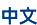## Algebra & Representation Theory

Algebras and symmetries occur throughout mathematics, science and engineering. It has been and remains one of the deepest and central areas in contemporary mathematics to understand the various complex algebraic structures and the different ways that a family of symmetries can arise.

The Langlands Program seeks a vast web of conjectures that connect automorphic representations and Galois representations, which is regarded as a grand unified theory, bridging together number theory, algebraic geometry, representation theory of associative algebras, and mathematical physics. One fundamental problem is to understand the infinite dimensional representations of reductive Lie groups, that involve families of continuous symmetries arising from finite dimensional geometries. Our research group in this area has made great progress with applications on related topics.

The research interests of this group include automorphic forms, representations of Lie groups and Lie algebras, cluster algebras and representations of associative algebras, Hopf algebras and quantum groups.

• 简介

Algebras and symmetries occur throughout mathematics, science and engineering. It has been and remains one of the deepest and central areas in contemporary mathematics to understand the various complex algebraic structures and the different ways that a family of symmetries can arise.

The Langlands Program seeks a vast web of conjectures that connect automorphic representations and Galois representations, which is regarded as a grand unified theory, bridging together number theory, algebraic geometry, representation theory of associative algebras, and mathematical physics. One fundamental problem is to understand the infinite dimensional representations of reductive Lie groups, that involve families of continuous symmetries arising from finite dimensional geometries. Our research group in this area has made great progress with applications on related topics.

The research interests of this group include automorphic forms, representations of Lie groups and Lie algebras, cluster algebras and representations of associative algebras, Hopf algebras and quantum groups.

• Liu Dongwen

Tenured Associate Professor

maliu@zju.edu.cn

• Wu Lei

Tenured Associate Professor

leiwu23@zju.edu.cn